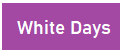Purple Days

Monday:

Learning Target: I can save a screen capture on my device.

Learning Target: I can make a Canvas Discussion forum post and insert in image in the post.

Learning target: I can write 3 forms of a linear function

Optional Graded work: In Canvas Complete the MOM Sec 2.1 – 2.3 assignment. Let me know if you want it to count. Due Monday 9/21

Required Graded Due Wednesday @ 2:30  in Canvas as a discussion post

1. Create two ordered pairs and graph them on paper
2. Find the slope of the the line that passes through these points. Show the slope formula on that paper.
3. Write the equation of the line that passes through the points in
1. Slope – Intercept form
2. Standard form
3. Point – slope form
4. Add a point to your graph that is NOT on the first line.
1. Write the equation of the line, in point-slope form,  that is parallel to the first line and that passes through this new point.
2. Write the equation of the line, in point-slope form,  that is perpendicular to the first line and that passes through this new point
5. Write the equation of the vertical line that passes through the new third point.
6. Write the equation of the horizontal line that passes through the new third point.

Tuesday:

Learning Target: I can write a recursive and explicit definitions for an arithmetic sequence given and combination of the first term, the common difference, any two terms.

Notes and HO

In Canvas: Post your work to one of the paper exercises. Due Thursday 9/17

Canvas:MOM Arithmetic Sequences : Due Friday night by Midnight.

Wednesday – Friday: Get the work completed. Remember you can schedule help with me here https://bit.ly/2wkAtf5Monday-Tuesday-Wednesday: Lucky you no work to do

Thursday

Learning Target: I can save a screen capture on my device.

Learning Target: I can make a Canvas Discussion forum post and insert in image in the post.

Learning target: I can write 3 forms of a linear function

Optional Graded work: In Canvas Complete the MOM Sec 2.1 – 2.3 assignment. Let me know if you want it to count. Due Monday 9/21

Required Graded Due Monday @ 2:30  in Canvas as a discussion post

1. Create two ordered pairs and graph them on paper
2. Find the slope of the the line that passes through these points. Show the slope formula on that paper.
3. Write the equation of the line that passes through the points in
1. Slope – Intercept form
2. Standard form
3. Point – slope form
4. Add a point to your graph that is NOT on the first line.
1. Write the equation of the line, in point-slope form,  that is parallel to the first line and that passes through this new point.
2. Write the equation of the line, in point-slope form,  that is perpendicular to the first line and that passes through this new point
5. Write the equation of the vertical line that passes through the new third point.
6. Write the equation of the horizontal line that passes through the new third point.

Friday:

Learning Target: I can write a recursive and explicit definitions for an arithmetic sequence given and combination of the first term, the common difference, any two terms.

Notes and HO